Computer Science

# Lab 2 — Loops and Control With Bit

To begin, use PyCharm to create a project called `lab-2`. You should do all your work there.

Next, download lab-2-files.zip. This is a zip file, so you will need to extract its contents. You should put the files inside of a folder called `lab-2` in your CS 110 folder. These files contain worlds. A world is a file that contains a description of what Bit sees.

You should have a folder called `lab-2/worlds/`, and then a bunch of files in there.

You are going to write your code in the `lab-2/` folder.

## Run to the Lake!

Bit: “How do I get to the lake?”

Helpful passerby: “Take a left a the stop sign.”

For this problem, make Bit do the following:

• go straight until it reaches a red square
• turn left
• go straight until it reaches a blue square
• paint all squares green, except those that are red or blue.

Use the file `go-to-lake-start.txt` as the starting world for Bit. You can load this file with:

``bit = Bit.load("worlds/go-to-lake-start.txt")``

When you start, the world looks like this: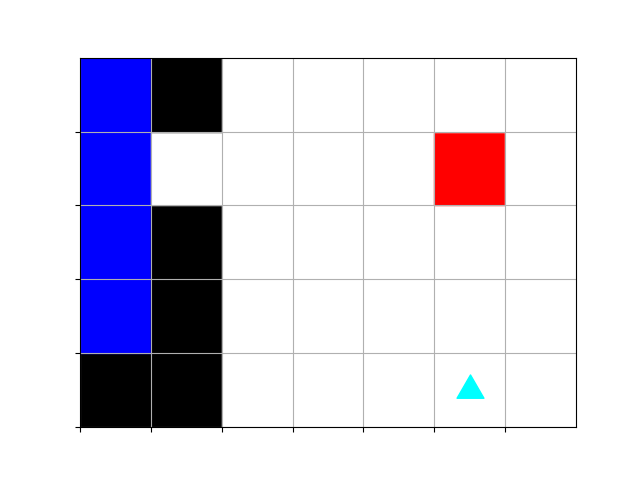When you finish, the world should look like this: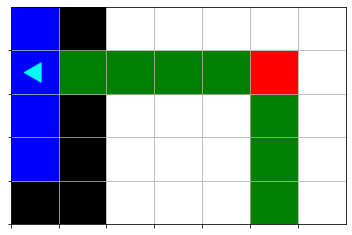Create a file called `lake.py` and write your code there. You should by loading the world:

``````from byubit import Bit

Once you are done, you can use this command to check your solution:

``bit.compare(Bit.load("worlds/go-to-lake-finish.txt"))``

There are many ways to solve this.

Try a solution with

``````while ...:
bit.paint("green")
bit.move()``````

and another solution with

``````while ...:
bit.move()
bit.paint("green")``````

Does one solution require more code than the other?

Which solution do you like more? Why?

## To Infinity…

Bit is trying to paint a square. It should look like this: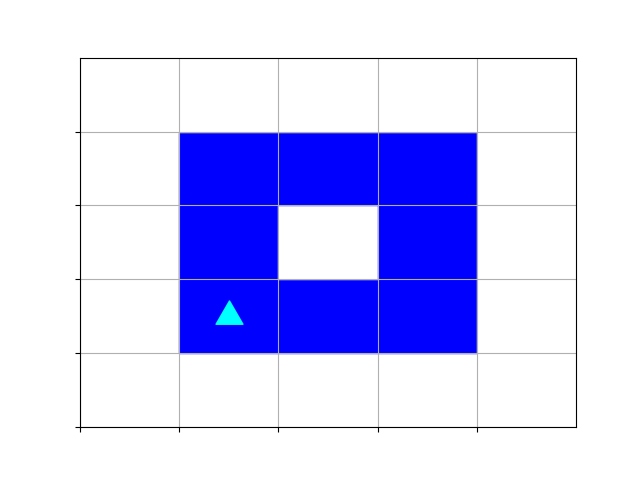But something isn’t working right! Run the code in `square.py`. What happens?

## Roofing the House

Help Bit build a roof for his house.

The roof should be red. It can extend 3 squares beyond a truss. If there is another truss under the third square, the roof can continue to extend.

Write your code in a file called `roofing.py`. Here is a starting world, in `roofing-start.txt`: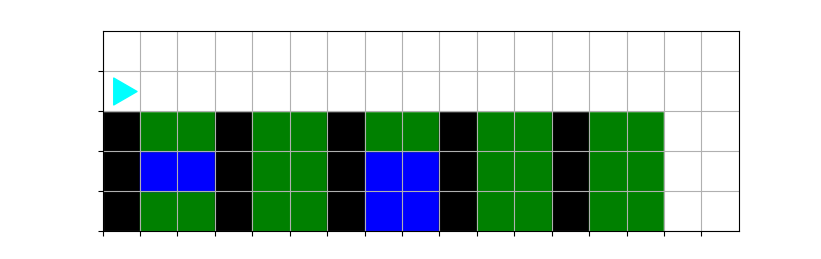The trusses are shown in black.

Remember, you can load this with:

``````from byubit import Bit

Now implement your solution, which should look like this when you are done: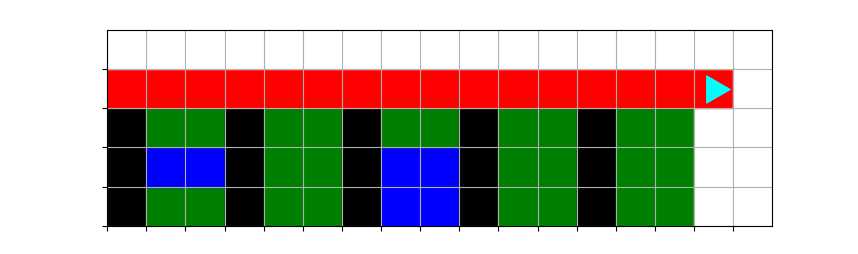You can compare your solution with:

``bit.compare(Bit.load("worlds/roofing-finish.txt"))``

## Observation

For a loop to continue iterating, the condition of the loop does not need to be true the whole time the body of the loop is running. It only needs to be true when the condition is evaluated.

For example, consider the following `while` loop:

``````while bit.get_color() == "blue":
bit.move()
bit.move()``````

If Bit starts on a `"blue"` square, then Bit will move twice: once from the first `"blue"` square to the second square, and once from the second square to the third. If the third square is `"blue"`, then the loop will repeat, but the second square could be any color!

🧐 It is important to remember that the condition of a `while` loop is only checked at the beginning of the loop body. Then the body of the loop is executed. Finally, the whole process repeats until the loop condition is false.

## Not too far!

### Look where you are going

Bit code cannot just do a `move()` any old time. If Bit moves into a black area, the move is blocked and the program returns an error. To prevent this, each a move needs to be preceded by a `front_clear()` check.

### Double Move

The goal of this problem is to paint every other square red in a row (the first square clear, the second square red). You ignore the first square. Here is an example of a 9x3 world: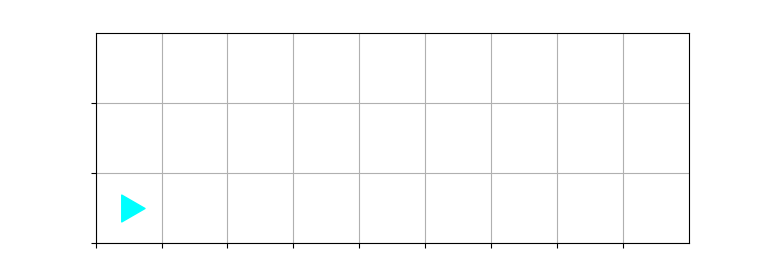Write your code in a file called `double-red.py`. You can create this world with:

``````from byubit import Bit
bit = Bit.new_world(9, 3)``````

At the finish, the world should look like this: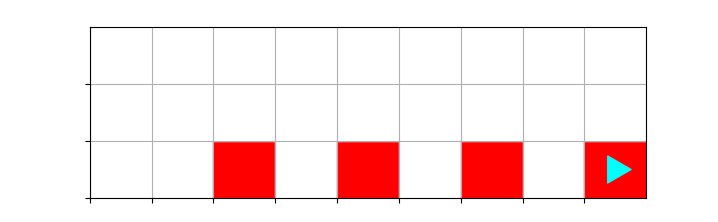You can compare your solution with:

``bit.compare(Bit.load("worlds/double-red-finish.txt"))``

Does your same code work for a 10x3 world?

``````from byubit import Bit
bit = Bit.new_world(10,3)``````

It should draw this: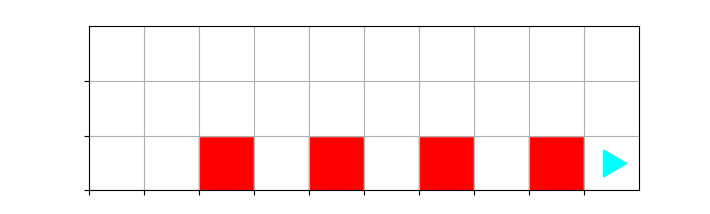You can compare your solution with:

``bit.compare(Bit.load("worlds/double-red2-finish.txt"))``

## #Tip

An interesting part of this problem is that Bit needs to move twice — once for the blank square and once for the red one. The first move is covered by the `front_clear()` check, but the second move is not.

We can solve this with an `if` statement. Add an `if` statement before the second move, and make the second move conditional on the `if` statement.

The `while` condition protects the first move, and `if` the front is still clear, Bit can make the second move.

Be sure your code works on both scenarios.

## Invert

Change every empty sqaure to blue and every blue sqaure to empty.

Note, you can erase a color using `bit.erase()`

Write your code in a file called `invert.py`. Start the world with the file called `invert-start.txt`. Follow our previous examples in this lab to load this world.

The world starts like this: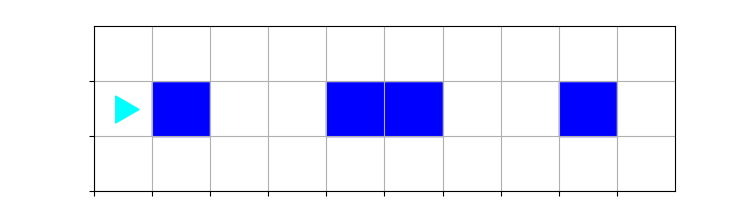and should end like this: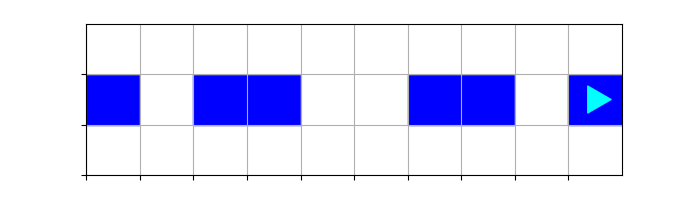You can compare with:

``bit.compare(Bit.load("worlds/invert-finish.txt"))``

## Invert…Carefully

For this problem, the rules are:

• change blue squares to empty
• change empty squares to blue
• leave everything else alone

Write your code in a file called `invert2.py`. The starting world is in the file `invert2-start.txt` and looks like this: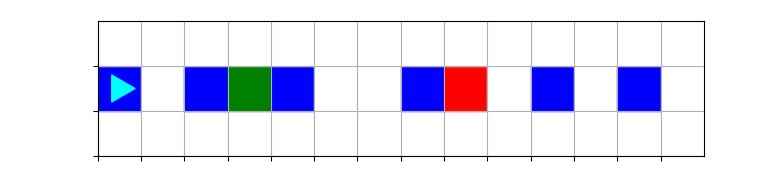At the end, it should look like this: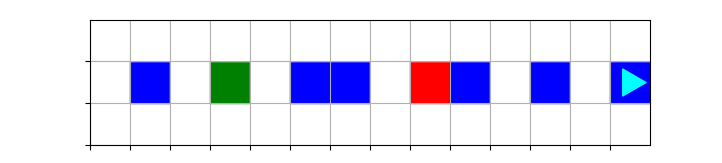You can compare your solution with:

``bit.compare(Bit.load("worlds/invert2-finish.txt"))``

### if, elif, and else

`if` and `else` work when you have two outcomes to pick from:

“Is my condition true? Yes: do if-block. No: do else-block.”

What if you have more than two outcomes? For example, consider the case below where we are trying to move Bit to a space that is clear.

``````if bit.front_clear():
bit.move()

elif bit.left_clear():
bit.left()
bit.move()

elif bit.right_clear():
bit.right()
bit.move()

else:
pass  # Bit is trapped, so nothing to do``````

We use `elif` to mean “else if”, mashing “else” and “if” together.

It’s also important to note that Python doesn’t like empty blocks for `if` or `while`. In this case, you can use `pass` if you have a block that doesn’t need any code.

### Checking for an empty square

What color is an empty square? It’s `None`. So you can check for this with:

``if bit.get_color() is None:``

## Bit the Wanderer

For this problem, the starting world is in `wander-start.txt` and looks like this: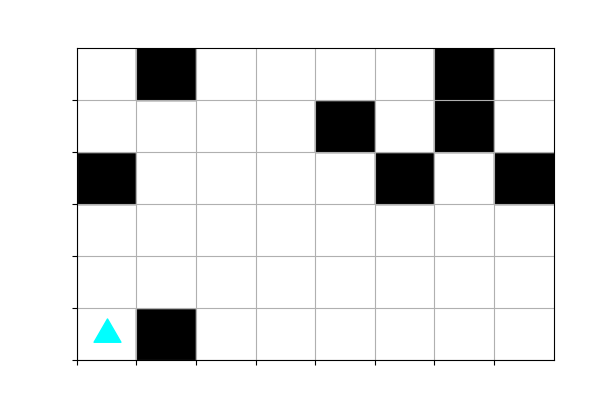The rules for bit are:

• paint the starting block green
• move Bit forward when you can
• paint new blocks blue
• if Bit is blocked in front, turn in the direction that is clear
• if Bit is blocked in every direction, stop
• paint the ending block red

The finished world should look like: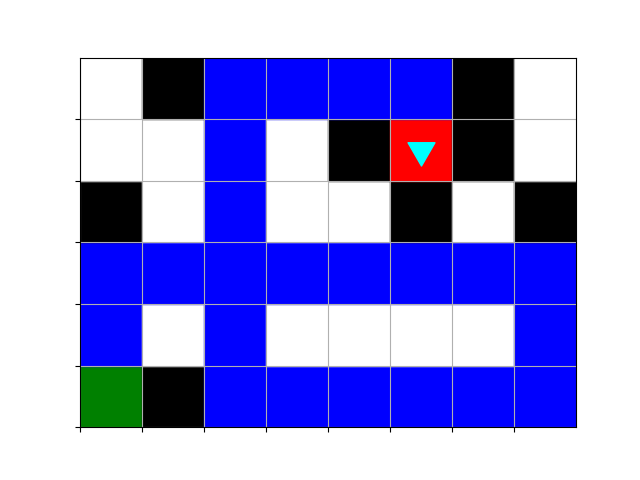You can compare your result with:

``bit.compare(Bit.load("worlds/wander-finish.txt"))``

You’ll need a few new bricks in your bag for this.

### Multiple conditions

When you want to test for whether two or more conditions are all true, use `and`:

``````while bit.front_clear() and bit.left_clear() and bit.right_clear():
...``````

If you want to test whether any of a set of conditions are true, use `or`:

``````while bit.front_clear() or bit.left_clear() or bit.right_clear():
...``````

### Nested loops

You will also want to use nested loops.

• The outer loop is controlled by condition A
• The inner loop is controlled by condition B
``````while <condition A>:
# May need code here?
while <condition B>:
# Will definitely need code here``````

❗️Remember, indentation matters!

The inner loop will have more indentation than the outer loop.

### Strategy:

• What is the condition of the outer loop?
• How do we know our solution is complete?
• What are the two pieces of the outer loop body?
• Go until…
• “go until” sounds like it will need a loop.
• What is the condition of this loop?
• Then make a decision…
• “make a decision” sounds like it will need an `if`.
• What is the condition to test?

## Lessons

What we want you to get from this lab:

• You feel confident writing while loops and choosing the expression for the while loop

• You feel confident writing if statements and choosing the conditions for the if statements

• You know when to use a while loop and when to use an if statement

• You can think carefully about what is needed before you write your code

• You can figure out what went wrong when something unexpected happens